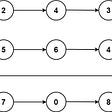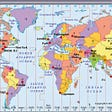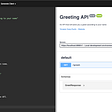# Solution

`var top = matrix[layer][i];var right = matrix[i][length - layer];var bot = matrix[length - layer][length -i];var left = matrix[length - i][layer];`
• Top will be fixed the row, only the column moving by i
• Right fixed by the column, only the row moving by i
• Bottom fixed by the row, opposite of the top
• Left fixed by the column, opposite of the right
`// top = leftmatrix[layer][i] = left;// right = topmatrix[i][length - layer] = top;// bot = rightmatrix[length - layer][length - i] = right;// left = botmatrix[length - i][layer] = bot;`
`public class Solution {    public void Rotate(int[][] matrix) {        var layers = matrix.Length / 2;        var length = matrix.Length - 1;                for(var layer = 0; layer < layers; layer++){                        for(var i = layer; i < length - layer; i++){                var top = matrix[layer][i];                var right = matrix[i][length - layer];                var bot = matrix[length - layer][length -i];                var left = matrix[length - i][layer];                                    // top = left                matrix[layer][i] = left;                // right = top                matrix[i][length - layer] = top;                // bot = right                matrix[length - layer][length - i] = right;                // left = bot                matrix[length - i][layer] = bot;            }        }    }}`

--

--

--

## More from Finn Nguyen

Software Engineer who is highly interested in building high scalability systems.

Love podcasts or audiobooks? Learn on the go with our new app.

## GSSOC : Open Source….a journey not a destination..!!## Building a secure PST cross-chain verification system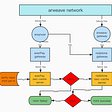## I am a Japanese Software Engineer.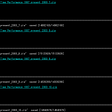## Data Pipeline Solution — Part 2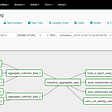## Distributed Tracing with Azure Spring Cloud and Azure Application Insights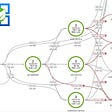## Aligned Dev Blog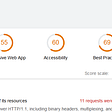## Finn Nguyen

Software Engineer who is highly interested in building high scalability systems.

## More from Medium